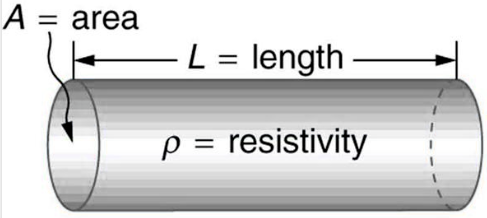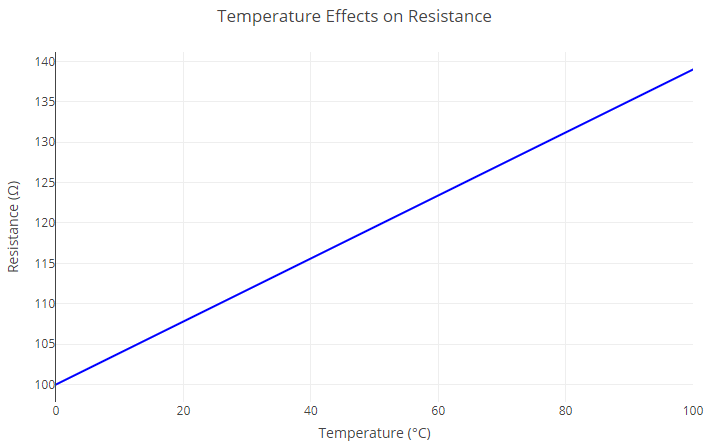## Resistance

Last updated on 2023-04-23 4 mins. to read

Electrical resistance measures a material's opposition to the flow of electric current. It is essential to understand the behaviour of electrical circuits and electronic devices. Electrical resistance is denoted by the symbol "R" and is measured in ohms (Ω).

## Factors Affecting Electrical Resistance

Several factors affect electrical resistance, including the following:

• Material: Different materials have different electrical resistivities. For example, copper has a low electrical resistivity, while rubber has a high electrical resistivity.
• Length: The longer the wire or conductor, the higher its resistance.
• Cross-sectional Area: The larger the wire or conductor, the lower its resistance.
• Temperature: The resistance of a material increases as its temperature increases.

## Calculating Electrical Resistance

The electrical resistance of a material can be calculated using the following formula:

$R=\frac{\rho \cdot L}{A}$

Where:

• R = electrical resistance (in ohms)
• ρ = resistivity of the material (in ohm-meters)
• L = length of the wire or conductor (in meters)
• A = cross-sectional area of the wire or conductor (in square meters)Resistivity, area and length

This formula shows that the resistance of a material is directly proportional to its length and resistivity and inversely proportional to its cross-sectional area. Therefore, longer wires or conductors made of materials with high resistivity will have higher resistance, while larger wires or conductors made of materials with low resistivity will have lower resistance.

### Example:

A copper wire has a length of 10 meters and a cross-sectional area of 0.5 square millimetres. The resistivity of copper is 1.68 x 10-8 ohm-meters. What is the electrical resistance of the wire? Using the formula, we can calculate the electrical resistance of the copper wire:

$R=\frac{1.68{10}^{-8}L}{A}==3.36\Omega$

Therefore, the electrical resistance of the copper wire is 3.36 ohms.

## Temperature Effects on Electrical Resistance

The resistance of a material increases as its temperature increases. This phenomenon is known as the temperature coefficient of resistance and is denoted by the alpha (α) symbol. The temperature coefficient of resistance is a measure of how much the resistance of material changes with temperature and is given by the following formula:

$\alpha =\frac{1}{R}×\frac{\mathrm{dR}}{\mathrm{dT}}$

Where:

• α = temperature coefficient of resistance (per degree Celsius)
• R = electrical resistance of the material at a reference temperature
• dR/dT = rate of change of resistance with respect to temperature

This formula assumes a linear relationship between resistance and temperature over the given temperature range.Variation of resistance with temperature

For most materials, the temperature coefficient of resistance is positive, which means that the resistance increases with temperature. However, some materials, such as carbon and silicon, have a negative temperature coefficient of resistance, which means their resistance decreases with temperature.

The formula for calculating electrical resistance due to temperature change is:

$R={R}_{0}\left[1+{\alpha }_{20}\left({T}_{2}-{T}_{1}\right)\right]$

Where:

• R is the electrical resistance of the material at a given temperature (T).
• R0 is the electrical resistance of the material at a reference temperature (T0).
• α is the temperature coefficient of resistance.
• T1 is the given reference temperature in degrees Celsius
• T2 is the given temperature in degrees Celsius